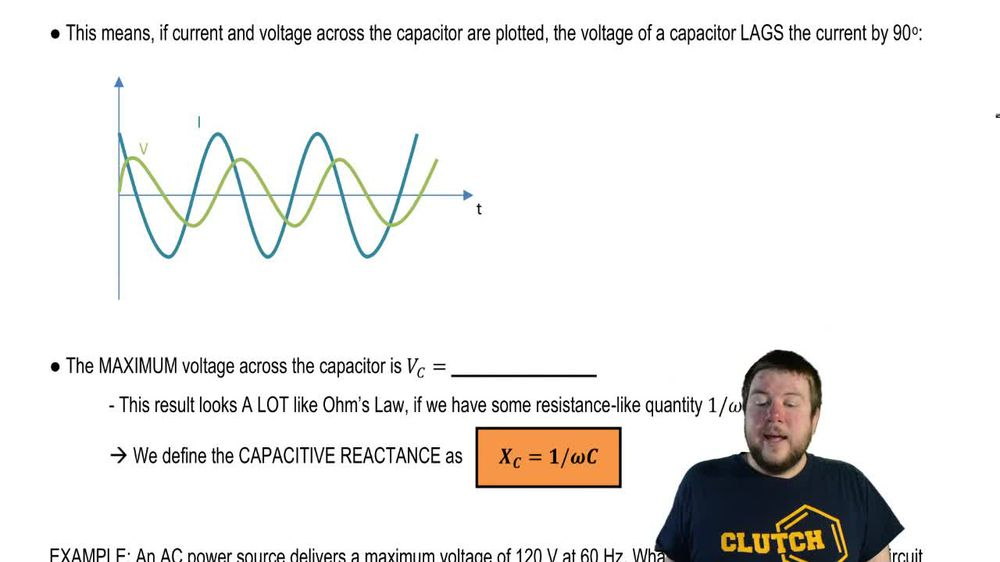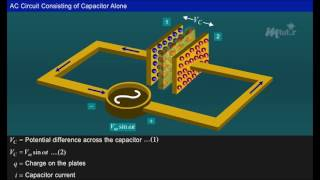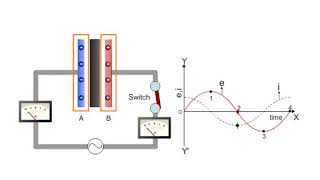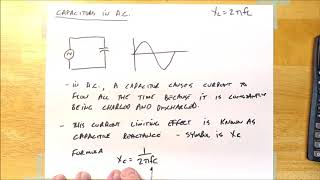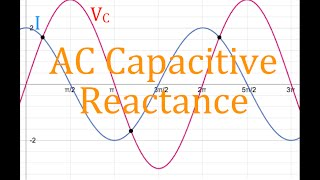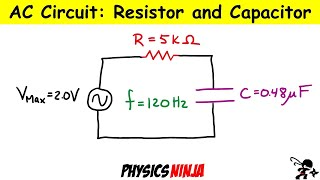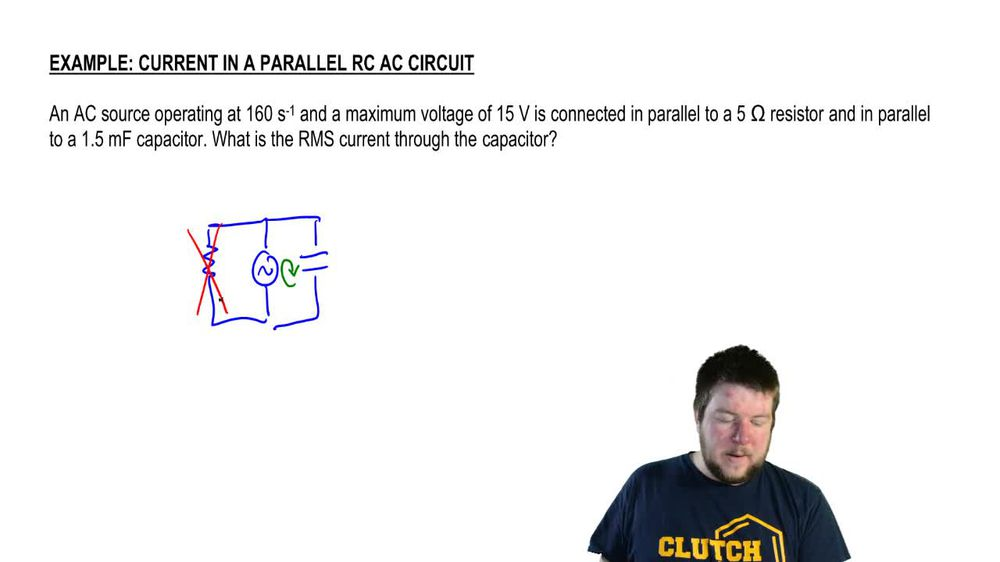Start typing, then use the up and down arrows to select an option from the list.
1. 31. Alternating Current2. Capacitors in AC Circuits# Capacitors in AC Circuits

by Patrick Ford
134 views
3
0
Hey, guys, in this video, we're gonna talk about the roles that capacitors play in a sea circuits. All right, let's get to it now. The current Macy's circuit, as we've seen before, is always given by Max Times CoSine of Omega T. Okay, something that you guys need to remember is that the voltage across the capacitor is always gonna be the charge of the capacitor at that particular incident time divided by the capacitance. This is from all the way back when we talked about D. C circuits. Okay, now there's a relationship between the amount of charge on a capacitor and the current in the circuit, and this is something that we've seen before. Now, to find that relationship, we have to use calculus. But I'll just show you the end result. The end result is that the charge as a function of time looks like this. So if I divide that charge as a function of time by the capacitance, I can say that the voltage across capacitor in an A C circuit at any time is gonna be Q divided by Omega Psi. Sorry. Not cute. I'm Max, divided by omega Psi times. Cosine of Omega T minus pi over two. Now, this is interesting, because when we saw the voltage across the resistor as a function of time, we saw that it was imax. Times are times cosine of omega t. So the angle off the co sign right this angle equals omega T is different than the angle for the voltage across the capacitor. This new angle, which I'll call data prime, is Omega T minus pi over two. That means that their functions, the current in the circuit and the voltage across the capacity are not gonna line up. Okay. When I plot them together, you'll see that they don't line up. In fact, the voltage of the capacitor lags the current by degrees. So if you look at any point in time right here, what is the current doing? The current is dropping, But what is the voltage doing? The voltage is at a maximum, right? But then it wants to match what the current is doing. So then, at a later time, it starts dropping. But at that time, the current has already balanced out. Okay, then at a later time, the voltage matches. What the current, Doesn't it balances out, but at this point, the current is already on the rise. So you see, the current is leading the voltage. The voltage is just trying to catch up and match what the current is doing. But the current leads it, or we say that the voltage lags the current. Okay. Another thing to notice is that the maximum voltage across the capacitor was just the amplitude of that equation. It was just imax divided by Omega Psi. This looks a lot like OEMs law. Remember that owns law for resistor says that the voltage across the resisters I times the resistance. Well, this looks like the current times that some quantity one overall magazine right? I can rewrite. This is I Max times one over Omega Psi. So one overall, Negus e looks like a resistance like quantity. It's a resistance like quantity. It carries the units off OEMs and we call it the capacitive reactant. Okay, so it's the reactant in a reactant acts like a resistance. Okay. And the capacitive reactant is one over Omega psi. Okay, so let's do an example, And a C power source delivers a maximum voltage off 120 volts at 60 hertz. What is the maximum current in a circuit with this power source connected to a 100 micro Farhad capacitor. Okay, so we want to know the maximum current in the circuit. We know the maximum current and a capacitor circuit is going to be given by I. Max equals the maximum voltage across the capacitor, divided by the capacitive reactant. Okay, that's just using this equation right here. Now, the question is, what is the capacity of reactant since? Or the capacity of reactant is one over omega times to capacitance. Omega is an angular frequency. We're told a linear frequency, so we have to convert the two first. Okay, remember that omega is defined is two pi f, which is two pi times 60 which is 377 in verse seconds. Okay, so now we confined the capacitive reactant, which is one over 3 times 100 micro is 10 to the negative six fair ads, and this equals 26 5. Remember that the units of reactant our homes because they are a resistance like quantity. Finally, the maximum currents, which is just the maximum voltage over the capacitor divided by the capacity of reactant. Since what is the maximum voltage across the capacitor? Okay, the capacities connected directly to the battery. So it has to share the maximum voltage with the battery. Right? That's just care. Jobs loop rule. Okay, the maximum voltage of the battery is 120 volts. So this is 1 20 divided by the capacity of reactions, which is 26.5. And that's gonna be 453 amps. Okay. Alright, guys, that wraps up our discussion of capacitors in a sea circuits. Thanks for watching.